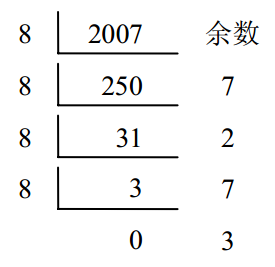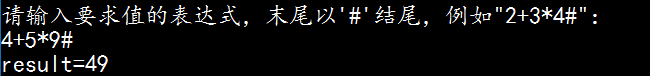# 栈的应用举例

## 数制转换应用：

N = (N div d) * d + N mod d(其中：div为整除运算，mod为求余运算)### 程序代码：

//conversion.h
/*
可以进行数制的转换功能
十进制转换位其他进制数，这能转化为10进制以内进制
*/

void conversion(){
SqStack S;
int N,M,e;
InitStack_Sq(S);
cout<<"请输入要转换的数字"<<endl;
cin>>N;
cout<<"请选择要转换的进制数（例如二进制，输2,只能转化为10进制以内的）："<<endl;
cin>>M;
while(N){
Push_Sq(S,N%M);
N=N/M;
}
cout<<N<<"进制的数转化为"<<M<<"进制后为：";
while(!StackEmpty_Sq(S)){
Pop_Sq(S,e);
cout<<e;
}
cout<<endl;

## 表达式求值算法：

4+（6-10+2*2）*21

• （1）先乘除，后加减；
• （2）从左算到右
• （3）先括号内，后括号外

4+（6-10+2*2）*2 = 4+（-4+2*2）*2 = 4+（-4+4）*2 = 4+0*2 = 4+0 = 41

• θ1 < θ2，θ1的优先权低于θ2
• θ1 = θ2，θ1的优先权等于θ2
• θ1 > θ2，θ1的优先权大于θ2

θ1 \ θ2+-*/()
+>><<<>
->><<<>
*<<>><>
/<<>><>
(<<<<<=
)>>>>>

• （1）首先置操作数栈和操作符栈为空栈

• （2）依次读入表达式中的每个字符，若是操作数则进OPND栈，若是运算符则和OPTR栈的栈顶运算符比较优先权后作相应操作。

1.若该运算符优先权大于栈顶运算符优先权则该运算符直接进OPTR栈；反之若运算符优先权小于栈顶运算符的优先权，则弹出栈顶运算符，并从操作数OPND栈弹出两个数进行该栈顶运算符的运算，运算结果再加入OPND操作数栈。
2.循环往复地进行第1步判断。直到将该操作符加入OPTR操作符栈

• （3）表达式读取结束，若两个栈都不为空，则依次弹出OPTR栈中的运算符和OPND栈中的两个操作数，进行运算后，将运算结果在加入OPND栈。直到OPTR栈为空，OPND栈只剩下一个元素，则该元素就是运算结果。

• （4）中间出现差错，比如最后OPND栈剩下不止一个数，则视为表达式出错。

### 程序代码:

//EvaluateExpression.h
//表达式求值算法

typedef int OperandType; //运算数类型
//------判断两个运算符的优先级-----------

char Precede(char theta1, char theta2){
//“#”是表达式的结束符，表达式的最左边也需设一个"#"构成表达式的一对括号
char theta = {'+', '-', '*', '/', '(', ')', '#'}; //定义操作符数组
//优先级比较数组
char prior={
{'>','>','<','<','<','>','>'},
{'>','>','<','<','<','>','>'},
{'>','>','>','>','<','>','>'},
{'>','>','>','>','<','>','>'},
{'<','<','<','<','<','=','0'},
{'>','>','>','>','0','>','>'},
{'<','<','<','<','<','0','='}};
int i = 0, j = 0;//i和j分别为两个操作符在数组中的位置
while( i < 7 && j < 7){
if(theta1!=theta[i]) i++;
if(theta2!=theta[j]) j++;
if(theta1 == theta[i] && theta2 == theta[j]) break;
}
return prior[i][j];//返回相应的优先级
}//Precede

//------根据给出的操作数op1和op2，以及操作符theta得出运算结果---------
OperandType Operate(OperandType op1, char theta, OperandType op2){
switch(theta){
case '+':
return op1+op2;
case '-':
return op1-op2;
case '*':
return op1*op2;
case '/':
return op1/op2;
default:
return ERROR;
}
}//Operate

//------------判断字符e是否为操作符----------------
Status In(char e, char *op){
int i = 0;
while(i++ < 7){
if(e==(*op++))
return TRUE;
}
return FALSE;
}//In

/*
算数表达式求值的算符的优先算法，设OPTR和OPND分别为运算符栈和运算符数栈，
测试时表达式必须以“#"结尾，如"2+3*5#"-------------------------------
这里为了算法简单，不考虑多数据类型，测试时均为一位整数。
*/
OperandType EvaluateExpression(){
char op={'+', '-', '*', '/', '(', ')', '#'};//op为运算符集合
SqStack OPTR,OPND;
InitStack_Sq(OPTR);
Push_Sq(OPTR,'#');
InitStack_Sq(OPND);
char c; //每次从键盘接收的字符
cin>>c;
SElemType e,x,theta; //e为每次进行判断的栈顶元素
SElemType a,b; //进行运算时栈顶取得的两个操锁数
SElemType result; //运算的最终结果
GetTop_Sq(OPTR,e); //获得数栈栈顶元素
while(c!='#' || e!='#'){
if(!In(c,op)){Push_Sq(OPND,c); cin>>c;}//不是运算符则进栈
else{
GetTop_Sq(OPTR,e);
switch(Precede(e,c)){
case '<': //栈顶元素优先权低
Push_Sq(OPTR,c);
cin>>c;
break;
case '=': //脱括号，并接收下一字符
Pop_Sq(OPTR,x);
cin>>c;
break;
case '>': //退栈并将运算结果入栈
Pop_Sq(OPTR,theta);
Pop_Sq(OPND,a);
Pop_Sq(OPND,b);
Push_Sq(OPND,Operate(b-48,theta,a-48)+48); //-48是将字符转化为数字
break;
}//switch
}//if
GetTop_Sq(OPTR,e);
}//while
GetTop_Sq(OPND,result);
cout<<"result="<<result-48<<endl;
return result-48;
}//Eva;uteExpression

### 程序运行结果：04-1905-031万+
02-071594
11-263524
03-319379
07-054060
06-09418
03-072975
04-02651
10-051971
07-29
11-20
12-122266
02-27441
06-141万+
05-17285
01-01126
08-16575
06-122269
©️2020 CSDN 皮肤主题: 技术工厂 设计师:CSDN官方博客